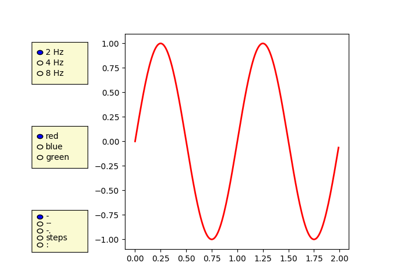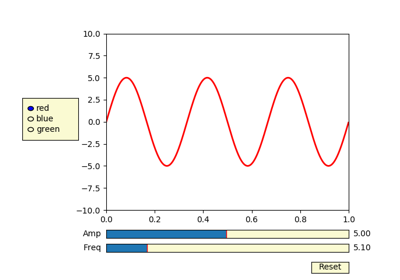# matplotlib.pyplot.axes¶

`matplotlib.pyplot.``axes`(arg=None, **kwargs)[source]

Add an axes to the current figure and make it the current axes.

Parameters:
arg : None or 4-tuple or Axes

The exact behavior of this function depends on the type:

• None: A new full window axes is added using `subplot(111, **kwargs)`

• 4-tuple of floats rect = `[left, bottom, width, height]`. A new axes is added with dimensions rect in normalized (0, 1) units using `add_axes` on the current figure.

• `Axes`: This is equivalent to `pyplot.sca`. It sets the current axes to arg. Note: This implicitly changes the current figure to the parent of arg.

Note

The use of an Axes as an argument is deprecated and will be removed in v3.0. Please use `pyplot.sca` instead.

Returns:
axes : Axes

The created or activated axes.

Other Parameters:
**kwargs :

For allowed keyword arguments see `pyplot.subplot` and `Figure.add_axes` respectively. Some common keyword arguments are listed below:

kwarg Accepts Description
facecolor color the axes background color
frameon bool whether to display the frame
sharex otherax share x-axis with otherax
sharey otherax share y-axis with otherax
polar bool whether to use polar axes
aspect [str | num] ['equal', 'auto'] or a number. If a number, the ratio of y-unit/x-unit in screen-space. See also `set_aspect`.

Examples

Creating a new full window axes:

```>>> plt.axes()
```

Creating a new axes with specified dimensions and some kwargs:

```>>> plt.axes((left, bottom, width, height), facecolor='w')
```

## Examples using `matplotlib.pyplot.axes`¶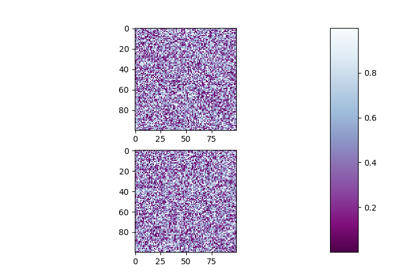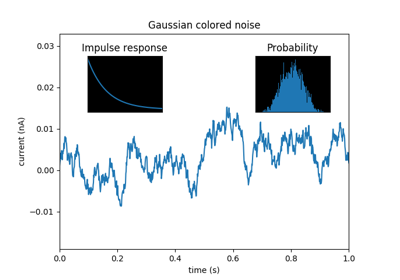Axes Demo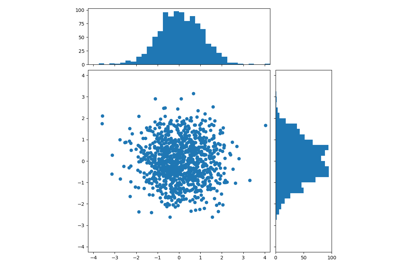Scatter Hist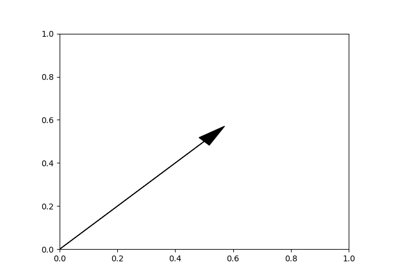Arrow Simple Demo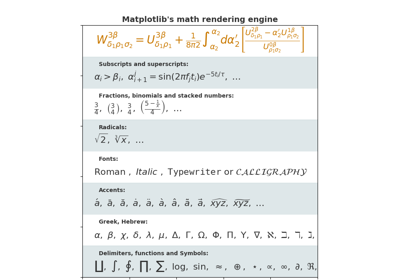Mathtext Examples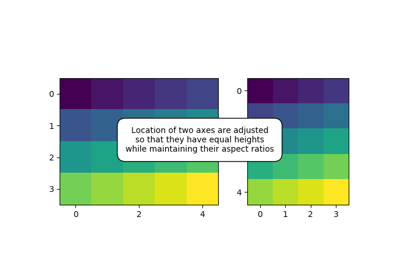Demo Axes Hbox Divider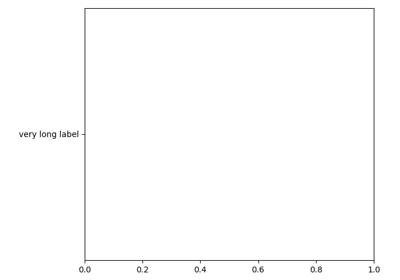Make Room For Ylabel Using Axesgrid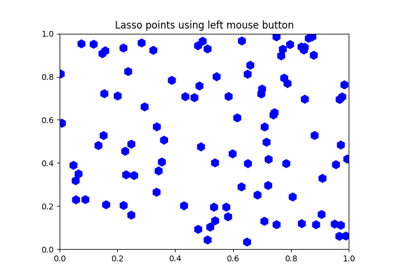Lasso Demo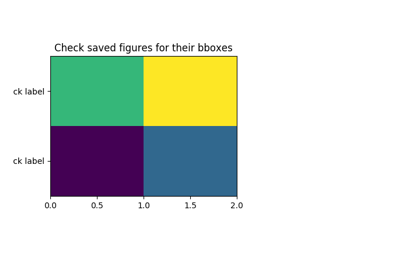Tight Bbox Test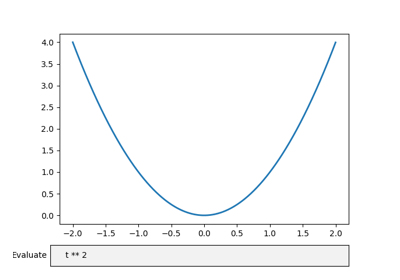Textbox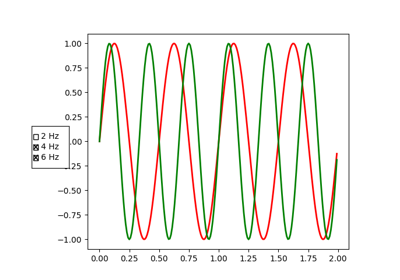Check Buttons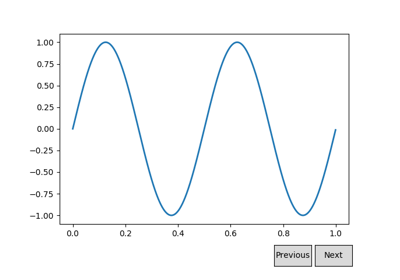Buttons